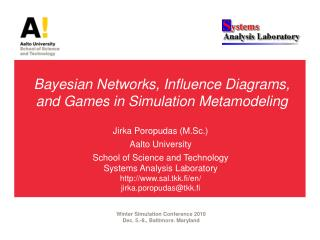DownloadDownload PresentationBayesian Networks, Influence Diagrams, and Games in Simulation Metamodeling

# Bayesian Networks, Influence Diagrams, and Games in Simulation Metamodeling

Télécharger la présentation## Bayesian Networks, Influence Diagrams, and Games in Simulation Metamodeling

- - - - - - - - - - - - - - - - - - - - - - - - - - - E N D - - - - - - - - - - - - - - - - - - - - - - - - - - -
##### Presentation Transcript

1. Bayesian Networks, Influence Diagrams,and Games in Simulation Metamodeling JirkaPoropudas (M.Sc.) Aalto University School of Science and TechnologySystems Analysis Laboratoryhttp://www.sal.tkk.fi/en/jirka.poropudas@tkk.fi Winter Simulation Conference 2010 Dec. 5.-8., Baltimore. Maryland

2. Contribution of the Thesis Novel Approaches to Simulation Metamodeling Simulation Metamodeling Time Evolution of Simulation Multiple Decision Makers with Individual Objectives Dynamic Bayesian Networks Decision Analysis with Multiple Criteria Games Influence Diagrams

3. The Thesis Consists of a summary article and six papers: Poropudas J., Virtanen K., 2010: Simulation Metamodeling with Dynamic Bayesian Networks, submitted for publication Poropudas J., Virtanen K., 2010: Simulation Metamodeling in Continuous Time using Dynamic Bayesian Networks, Winter Simulation Conference 2010 Poropudas J., Virtanen K., 2007: Analysis of Discrete Event Simulation Results using Dynamic Bayesian Networks, Winter Simulation Conference 2007 Poropudas J., Virtanen K., 2009: Influence Diagrams in Analysis of Discrete Event Simulation Data, Winter Simulation Conference 2009 Poropudas J., Virtanen K., 2010: Game Theoretic Validation and Analysis of Air Combat Simulation Models, Systems, Man, and Cybernetics – Part A: Systems and Humans, Vol. 40, No. 5 Pousi J., Poropudas J., Virtanen K., 2010: Game Theoretic Simulation Metamodeling using Stochastic Kriging, Winter Simulation Conference 2010 http://www.sal.tkk.fi/en/publications/

4. Dynamic Bayesian Networks and Discrete Event Simulation Simulation state at Bayesian network • Joint probability distribution of discrete random variables • Nodes • Simulation state variables Dependencies • Arcs • Conditional probability tables Dynamic Bayesian network • Time slices → Discrete time

5. DBNs in Simulation Metamodeling Time evolution of simulation • Probability distribution of simulation state at discrete times Simulation parameters • Included as random variables What-if analysis • Simulation state at time t is fixed→ Conditional probability distributions Poropudas J., Virtanen K., 2010. Simulation Metamodeling with Dynamic Bayesian Networks, submitted for publication.

6. Construction of DBN Metamodel Poropudas J., Virtanen K., 2010. Simulation Metamodeling with Dynamic Bayesian Networks, submitted for publication. Selection of variables Collecting simulation data Optimal selection of time instants Determination of network structure Estimation of probability tables Inclusion of simulation parameters Validation

7. Approximative Reasoningin Continuous Time • DBN gives probabilities at discrete time instants→ What-if analysis at these time instants • Approximative probabilities for all time instants with Lagrange interpolating polynomials → What-if analysis at arbitrary time instants ”Simple, yet effective!” Monday 10:30 A.M. - 12:00 P.M.Metamodeling I Poropudas J., Virtanen K., 2010. Simulation Metamodeling in Continuous Time using Dynamic Bayesian Networks, WSC 2010.

8. Air Combat Analysis Poropudas J., Virtanen K., 2007. Analysis of Discrete Events Simulation Results Using Dynamic Bayesian Networks, WSC 2007. Poropudas J., Virtanen K., 2010. Simulation Metamodeling with Dynamic Bayesian Networks, submitted for publication. X-Brawler ̶ a discrete event simulation model

9. Influence Diagrams (IDs) andDiscrete Event Simulation Poropudas J., Pousi J., Virtanen K., 2010. Simulation Metamodeling with Influence Diagrams, manuscript. Decision nodes • ”Controllable” simulation inputs Chance nodes • Uncertain simulation inputs • Simulation outputs • Conditional probability tables Utility nodes • Decision maker’s preferences • Utility functions Arcs • Dependencies • Information

10. Construction of ID Metamodel Poropudas J., Pousi J., Virtanen K., 2010. Simulation Metamodeling with Influence Diagrams, manuscript. Selection of variables Collecting simulation data Determination of diagram structure Estimation of probability tables Preference modeling Validation

11. IDs as MIMO Metamodels Poropudas J., Pousi J., Virtanen K., 2010. Simulation Metamodeling with Influence Diagrams, manuscript. Queueing model • Simulation parameters included as random variables • Joint probability distribution of simulation inputs and outputs • What-if analysis using conditional probability distributions

12. Decision Making with Multiple Criteria Decision maker’s preferences • One or more criteria • Alternative utility functions Tool for simulation baseddecision support • Optimal decisions • Non-dominated decisions

13. Air Combat Analysis Poropudas J., Virtanen K., 2009. Influence Diagrams in Analysis of Discrete Event Simulation Data, WSC 2009. Consequences of decisions Decision maker’s preferences Optimal decisions

14. Games andDiscrete Event Simulation Poropudas J., Virtanen K., 2010. Game Theoretic Validation and Analysis of Air Combat Simulation Models, Systems, Man, and Cybernetics – Part A: Systems and Humans, Vol. 40, No. 5, pp.1057-1070. Game setting Players • Multiple decision makers with individual objectives Players’ decisions • Simulation inputs Players’ payoffs • Simulation outputs Best responses Equilibrium solutions

15. Construction ofGame Theoretic Metamodel Poropudas J., Virtanen K., 2010. Game Theoretic Validation and Analysis of Air Combat Simulation Models, Systems, Man, and Cybernetics – Part A: Systems and Humans, Vol. 40, No. 5, pp.1057-1070. Definition of scenario Simulation data Estimation of payoffs • Regression model, stochastic kriging • ANOVA

16. Best Responses andEquilibirium Solutions Poropudas J., Virtanen K., 2010. Game Theoretic Validation and Analysis of Air Combat Simulation Models, Systems, Man, and Cybernetics – Part A: Systems and Humans, Vol. 40, No. 5, pp.1057-1070. Best responses ̶ player’s optimal decisions against a given decision by the opponent Equilibrium solutions ̶ intersections of players’ best responses

17. Games and Stochastic Kriging Tuesday 1:30 P.M. - 3:00 P.M.Advanced Modeling Techniques for Military Problems Extension to global response surface modeling Pousi J., Poropudas J., Virtanen K., 2010. Game Theoretic Simulation Metamodeling Using Stochastic Kriging, WSC 2010.

18. Utilization ofGame Theoretic Metamodes Validation of simulation model • Game properties compared with actual practices For example, best responses versus real-life air combat tactics Simulation based optimization • Best responses • Dominated and non-dominated decision alternatives • Alternative objectives